# Factor Analysis, Clustering, Hypothesis Testing, Linear Regression: Exercises and Solutions in R

In order to solve the tasks you need:

## Problem 1

Scenario: A survey was conducted to find out how teenagers think about the future and barriers they think will hinder their career. The researcher would like to determine if the 15 survey items can be summarized more efficiently by a smaller set of latent factors.

You will need these files:

• careerbarrier.rda: Data for the 15 survey items for a random sample of 76 teens.
• Career Barrier Survey.docx: Descriptions of the variables in the data file.

### Part A

#### Question 1

Conduct Bartlett’s test for sphericity on the responses for the 15 survey questions.

library(psych)
mat <- cor(careerbarrier)
cortest.bartlett(mat, n = 76)
## $chisq ##  287.4985 ## ##$p.value
##  6.756016e-19
##
## $df ##  105 #### Question 2 State the null and alternative hypothesis. $$H_0:$$ The correlation matrix is the identity matrix; $$H_a:$$ The correlation matrix is not the identity matrix. #### Question 3 State your conclusion at a 5% level of significance and respond with whether factor analysis is warranted based on this measure. Since p-value < 0.05, we reject the null hypothesis. The correlation matrix is not the identity matrix. Factor analysis is warranted based on this measure. #### Question 4 Round the p-value to four decimal places (enter 0 if P < 0.00005). p-value = 0 ### Part B1 #### Question 5 Compute the Kaiser-Meyer-Olkin (KMO) Measure of Sampling Adequacy (MSA) for the responses for the 16 survey questions. KMO(mat) ## Kaiser-Meyer-Olkin factor adequacy ## Call: KMO(r = mat) ## Overall MSA = 0.68 ## MSA for each item = ## money lazy law noexp math support health reading ## 0.36 0.67 0.67 0.61 0.67 0.66 0.67 0.73 ## english aoda grades disc social relatshp looks ## 0.69 0.82 0.67 0.66 0.75 0.72 0.58 #### Question 6 Report the overall MSA value. Overall MSA = 0.68 #### Question 7 Is the overall MSA value acceptable for factor analysis? Yes. #### Question 8 Should any questionnaire items be dropped from the factor analysis because of MSA values under 0.50? Yes. #### Question 9 If so which one(s)? (if there aren’t any, write “none”) Answer: money ### Part B2 #### Question 10 Compute the Kaiser-Meyer-Olkin (KMO) Measure of Sampling Adequacy (MSA) for the responses for the remaining survey questions after you have dropped the items from Part B1. Use the following questions to document your findings. mat2 <- cor(careerbarrier[, -1]) KMO(mat2) ## Kaiser-Meyer-Olkin factor adequacy ## Call: KMO(r = mat2) ## Overall MSA = 0.7 ## MSA for each item = ## lazy law noexp math support health reading english ## 0.68 0.67 0.61 0.68 0.66 0.68 0.74 0.70 ## aoda grades disc social relatshp looks ## 0.82 0.67 0.70 0.77 0.71 0.62 #### Question 11 Report the overall MSA value. Overall MSA = 0.7 #### Question 12 Is the new overall MSA value acceptable for factor analysis? Yes. #### Question 13 Should any questionnaire items be dropped from the factor analysis because of MSA values under 0.50? No. #### Question 14 If so which one(s)? (if there aren’t any say “none”) Answer: None. ### Part C #### Question 15 Use R to create a scree plot for the questionnaire items that you deemed to be appropriate for the factor analysis from Part B. output <- princomp(careerbarrier[, -1], cor = TRUE) plot(output, type = "lines", main = "Scree plot") abline(h = 1, lty = 2)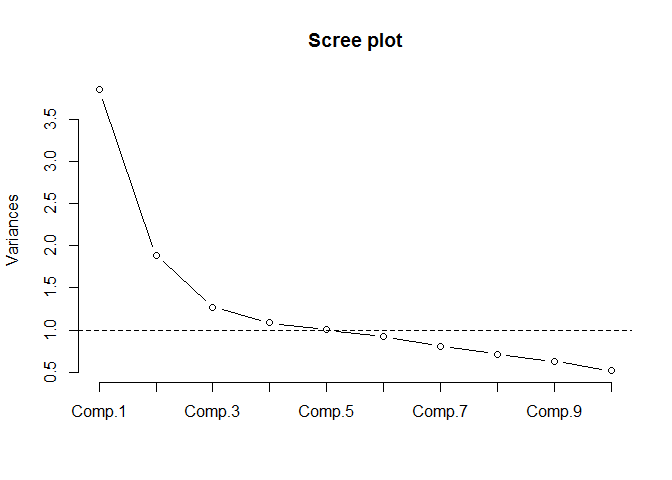#### Question 16 Using the knee in the scree plot, how many factors should be extracted? Answer: 3 #### Question 17 How many components have eigenvalues (aka variances, latent roots) greater than 1 and how many factors does this suggest extracting? Answer: 5 ### Part D #### Question 18 Use a principal components extraction with the varimax rotation to extract 5 factors. Print the output with factor loadings under 0.5 suppressed and sort the loadings. fa.out <- principal(careerbarrier[, -1], nfactors = 5, rotate = "varimax") print.psych(fa.out, cut = 0.5, sort = TRUE) ## Principal Components Analysis ## Call: principal(r = careerbarrier[, -1], nfactors = 5, rotate = "varimax") ## Standardized loadings (pattern matrix) based upon correlation matrix ## item RC2 RC1 RC3 RC4 RC5 h2 u2 com ## looks 14 0.79 0.67 0.33 1.2 ## relatshp 13 0.76 0.65 0.35 1.2 ## disc 11 0.71 0.53 0.47 1.1 ## social 12 0.59 0.50 0.50 1.9 ## reading 7 0.82 0.78 0.22 1.3 ## english 8 0.74 0.67 0.33 1.4 ## health 6 0.63 0.59 0.41 2.0 ## aoda 9 0.58 0.59 0.41 2.2 ## lazy 1 0.81 0.77 0.23 1.4 ## law 2 0.76 0.65 0.35 1.3 ## grades 10 0.65 0.53 0.47 1.5 ## support 5 0.82 0.71 0.29 1.1 ## math 4 0.64 0.60 0.40 2.0 ## noexp 3 0.89 0.85 0.15 1.1 ## ## RC2 RC1 RC3 RC4 RC5 ## SS loadings 2.28 2.28 2.11 1.27 1.15 ## Proportion Var 0.16 0.16 0.15 0.09 0.08 ## Cumulative Var 0.16 0.33 0.48 0.57 0.65 ## Proportion Explained 0.25 0.25 0.23 0.14 0.13 ## Cumulative Proportion 0.25 0.50 0.73 0.87 1.00 ## ## Mean item complexity = 1.5 ## Test of the hypothesis that 5 components are sufficient. ## ## The root mean square of the residuals (RMSR) is 0.08 ## with the empirical chi square 96.17 with prob < 1.4e-08 ## ## Fit based upon off diagonal values = 0.89 #### Question 19 What is the cumulative variance explained? Answer as a percent, not a decimal number. Answer: 65% #### Question 20 Is this considered an acceptable percent of total variation? Yes. ## Problem 2 Scenario: Water quality variables nitrogen, turbidity, phosphorus, dissolved oxygen, temperature, and conductivity are measured in 31 randomly selected farm ponds in Southeastern Minnesota. Researchers would like to determine if there is an underlying structure that will enable clustering of these 31 ponds into homogeneous groups. You will need this file: farmpondquality.rda ### Part A #### Question 21 Load the data set and standardize the variables in the file (i.e. find the z-scores for each value). Store the z-scores in a new data frame. load("farmpondquality.rda") farmpondquality_new <- scale(farmpondquality, center = TRUE, scale = TRUE) ### Part B #### Question 22 Plot the dendrogram for hierarchical clustering using complete linkage and add the rectangles by cutting the dendrogram at a height of 5. h_clusters <- hclust(dist(farmpondquality_new), method = "complete") plot(h_clusters) rect.hclust(h_clusters, h = 5)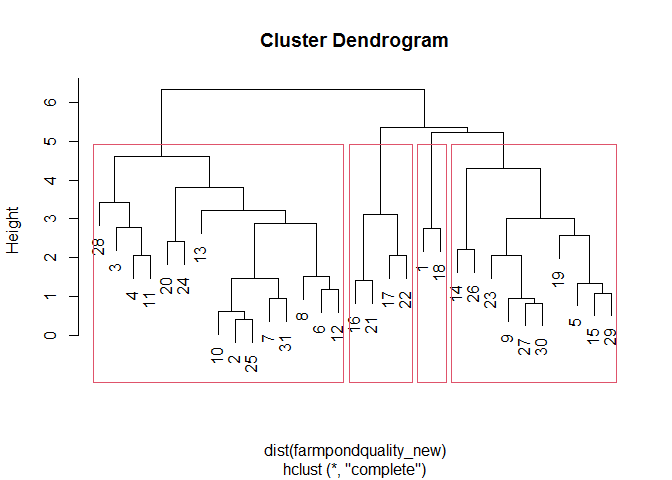#### Question 23 How many clusters does this form? 4 clusters. ### Part C #### Question 24 Append the original data frame (the unscaled one) with the cluster number from cutting the dendrogram at a height of 5. Find the number of ponds in each cluster and obtain the means of the original variables for each cluster. library(dplyr) # for easy grouping and summarising farmpondquality <- cbind(farmpondquality, h_clusters = cutree(h_clusters, h = 5)) tbl <- farmpondquality %>% group_by(h_clusters) %>% summarise(num_ponds = n(), mean_nitro = mean(nitro), mean_turb = mean(turb), mean_phos = mean(phos), mean_disoxy = mean(disoxy), mean_temp = mean(temp), mean_cond = mean(cond)) print.data.frame(tbl) ## h_clusters num_ponds mean_nitro mean_turb mean_phos mean_disoxy mean_temp ## 1 1 2 0.7865357 13.766667 2.5072143 9.510714 20.62500 ## 2 2 15 0.3006019 28.454413 1.8769083 9.224706 23.08413 ## 3 3 10 0.1819414 9.091905 1.6315357 11.507738 20.41036 ## 4 4 4 0.1832321 9.183929 0.9327321 8.553571 22.56696 ## mean_cond ## 1 346.7500 ## 2 149.6277 ## 3 206.7179 ## 4 488.5625 #### Question 25 For k-means clustering, plot the within sum of squares for the first 15 clusters against the cluster number and use the plot to determine a good number of clusters to partition the cases into. Use the standardized values. set.seed(123) wss <- (nrow(farmpondquality_new)-1) * sum(apply(farmpondquality_new, 2, var)) for (i in 2:15) { wss[i] <- sum(kmeans(farmpondquality_new, centers = i)$withinss)
}
plot(1:15, wss, type = "b",
xlab = "Number of Clusters",
ylab = "Within groups sum of squares")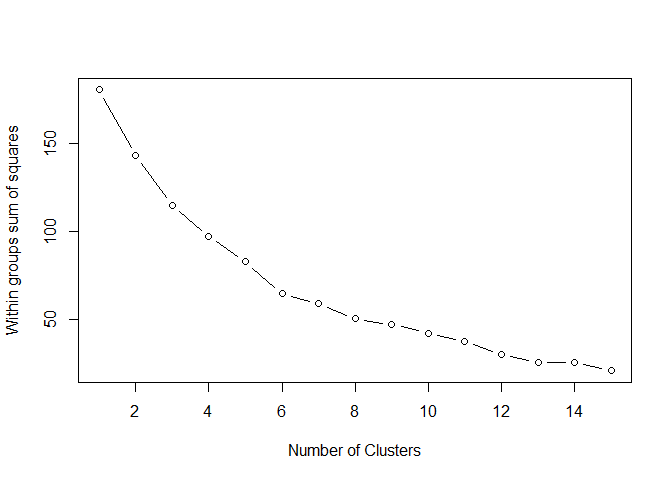#### Question 26

How many clusters do you think are best, based on this plot? Why?

6, because this is where the ‘knee’ seems to be in the plot (around 6 clusters, the decline slows down).

#### Question 27

Perform the k-means clustering on the z-scores of the 6 pond quality variables using the number of clusters you determined from the plot. Find the number of cases in each cluster as well as the cluster means for the raw variables.

k_means_cluster <- kmeans(farmpondquality_new, 6)
farmpondquality <- cbind(farmpondquality, k_means_clusters = k_means_cluster$cluster) tbl2 <- farmpondquality %>% group_by(k_means_clusters) %>% summarise(num_ponds = n(), mean_nitro = mean(nitro), mean_turb = mean(turb), mean_phos = mean(phos), mean_disoxy = mean(disoxy), mean_temp = mean(temp), mean_cond = mean(cond)) ## summarise() ungrouping output (override with .groups argument) print.data.frame(tbl2) ## k_means_clusters num_ponds mean_nitro mean_turb mean_phos mean_disoxy ## 1 1 4 0.6661321 11.833333 1.854054 9.031429 ## 2 2 5 0.1996857 8.747143 1.021886 9.097857 ## 3 3 6 0.2169155 15.863810 3.286909 11.557341 ## 4 4 3 0.4458810 48.966667 2.056605 5.532421 ## 5 5 2 0.2131071 52.107143 1.103179 9.171429 ## 6 6 11 0.1732851 13.148918 1.144643 10.980411 ## mean_temp mean_cond ## 1 22.40089 260.64375 ## 2 21.80357 471.90000 ## 3 22.08294 231.77579 ## 4 21.88690 172.33571 ## 5 24.76786 93.32143 ## 6 21.41526 132.99675 ## Problem 3 A study was conducted on the relationship of seating position and nausea on buses. The data in the following table classifies each person in a random sample of bus riders by the location of his or her seat and whether or not nausea was reported. TableFrontMiddleRearTotal Nausea98110161369 No Nausea264321280865 Total3624314411234 ### Part A #### Question 28 Test to see whether or not the seat position within a bus is associated with motion sickness. bus_riders <- matrix(c(98, 110, 161, 264, 321, 280), nrow = 2, byrow = TRUE) rownames(bus_riders) <- c("Nausea", "No Nausea") colnames(bus_riders) <- c("Front", "Middle", "Rear") bus_riders ## Front Middle Rear ## Nausea 98 110 161 ## No Nausea 264 321 280 chisq.test(bus_riders) ## ## Pearson's Chi-squared test ## ## data: bus_riders ## X-squared = 14.509, df = 2, p-value = 0.000707 #### Question 29 State the null and alternative hypothesis. $$H_0:$$ Motion sickness and seat position within a bus are independent variables; $$H_a:$$ Motion sickness and seat position within a bus are not independent variables. #### Question 30 State the test statistic. Give the answers to the nearest thousandth decimal. X-squared = 14.509 #### Question 31 State the degrees of freedom. df = 2 #### Question 32 Round the p-value to four decimal places (enter 0 if P < 0.00005). p-value = 0.0007 #### Question 33 State your conclusion. Since p-value < 0.05, we reject the null hypothesis that motion sickness and seat position within a bus are independent variables. The seat position within a bus is associated with motion sickness. ### Part B #### Question 34 Construct a 90% confidence interval (without the Yates correction) for the difference in population proportions of all bus riders in the front who report nausea and all bus riders in the rear who report nausea. (Use Diff = Front - Rear) bus_riders_tbl <- t(bus_riders[, -2]) bus_riders_tbl ## Nausea No Nausea ## Front 98 264 ## Rear 161 280 stats::prop.test(bus_riders_tbl, correct = FALSE, conf.level = 0.9) ## ## 2-sample test for equality of proportions without continuity ## correction ## ## data: bus_riders_tbl ## X-squared = 8.1012, df = 1, p-value = 0.004424 ## alternative hypothesis: two.sided ## 90 percent confidence interval: ## -0.14819088 -0.04053138 ## sample estimates: ## prop 1 prop 2 ## 0.2707182 0.3650794 #### Question 35 Enter the lower bound of the 90% CI (round to 3 decimal places). -0.148 #### Question 36 Enter the upper bound of the 90% CI (round to 3 decimal places). -0.041 #### Question 37 Write an interpretation for the interval in the context of the problem. We are 90% confident that the difference in population proportions of all bus riders in the front who report nausea and all bus riders in the rear who report nausea is between -0.148 and -0.041. ### Part C #### Question 38 Compute the odds ratio of having nausea for those in the rear compared to those in the front of the bus. library(mosaic) oddsRatio(bus_riders_tbl) ##  1.54898 #### Question 39 Report the odds ratio to 3 decimal places. 1.549 #### Question 40 Interpret the odds ratio in the context of the problem. For people sitting in the rear of the bus, the odds of having nausea are 1.549 times larger than the odds of having nausea for those in the front of the bus. ## Problem 4 Scenario : A random sample of apartments was obtained from mid-sized towns in the Midwest. They are classified as having either “3 bedrooms” or “2 bedrooms” and the monthly rent was recorded. You will need this file: rent.rda ### Part A #### Question 41 Load the data set and create boxplots for the monthly rents for each type of apartment. load("rent.rda") boxplot(rent ~ type, data = monthlyrent, xlab = "Type", ylab = "Rent")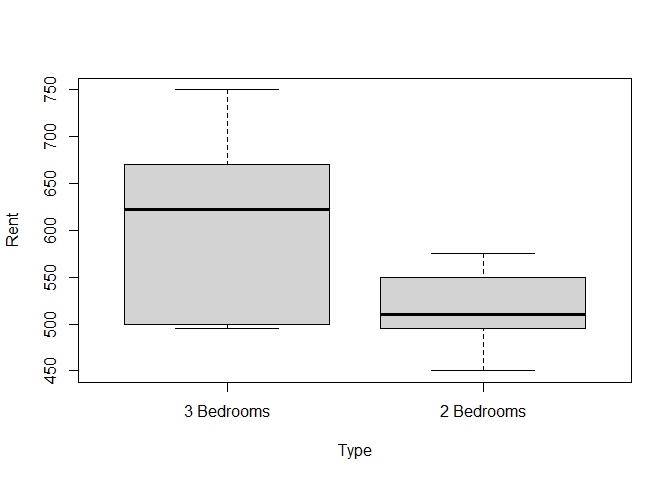#### Question 42 Comment on the shapes of the boxplots and whether or not they contain outliers. Does there appear to be a difference in the distributions of monthly rent between 2 and 3 bedroom apartments? The box for 3 bedroom apartments is longer than the box for 2 bedroom apartments and the distribution for 3 bedroom apartments seems to be skewed to the left, while the distribution for 2 bedroom apartments seems to be skewed to the right. There are no outliers. There appears to be a difference in the distributions of monthly rent between 2 and 3 bedroom apartments. ### Part B #### Question 43 Conduct the Shapiro-Wilk test for normality for each sample. shapiro.test(monthlyrent$rent[monthlyrent$type == "3 Bedrooms"]) ## ## Shapiro-Wilk normality test ## ## data: monthlyrent$rent[monthlyrent$type == "3 Bedrooms"] ## W = 0.90679, p-value = 0.2596 shapiro.test(monthlyrent$rent[monthlyrent$type == "2 Bedrooms"]) ## ## Shapiro-Wilk normality test ## ## data: monthlyrent$rent[monthlyrent$type == "2 Bedrooms"] ## W = 0.96512, p-value = 0.8423 #### Question 44 Using a 5% level of significance for each test individually, choose the option that describes conclusions for each distribution. 1. Normality is rejected for the rents of both the 3-bedroom and 2-bedroom apartments. 1. Normality is rejected for the rents of the 2-bedroom apartments but not the 3-bedroom apartments. 1. Normality is rejected for the rents of the 3-bedroom apartments but not the 2-bedroom apartments. 1. Normality is not rejected for the rents of either the 3-bedroom or 2-bedroom apartments. Answer: (d) Normality is not rejected for the rents of either the 3-bedroom or 2-bedroom apartments. ### Part C #### Question 45 Test for equality of population variances using a 5% level of significance. var.test(rent ~ type, data = monthlyrent) ## ## F test to compare two variances ## ## data: rent by type ## F = 5.2305, num df = 9, denom df = 9, p-value = 0.02159 ## alternative hypothesis: true ratio of variances is not equal to 1 ## 95 percent confidence interval: ## 1.299192 21.058128 ## sample estimates: ## ratio of variances ## 5.230541 #### Question 46 State the null and alternative hypotheses. $$H_0:$$ The variances of the rents of both the 3-bedroom and 2-bedroom apartments are equal; $$H_a:$$ The variances are not equal. #### Question 47 Round the p-value to four decimal places (enter 0 if P < 0.00005). p-value = 0.0216 #### Question 48 State the conclusion for the test. Since p-value < 0.05, we reject the null hypothesis. The variances of the rents of the 3-bedroom and 2-bedroom apartments are not equal. ### Part D #### Question 49 Conduct the appropriate hypothesis test (for two samples only - not ANOVA) to compare the population mean rents for these two types of apartments in mid-sized Midwestern towns. Use a 10% level of significance. t.test(rent ~ type, data = monthlyrent, paired = FALSE, var.equal = FALSE, conf.level = 0.9) ## ## Welch Two Sample t-test ## ## data: rent by type ## t = 3.0105, df = 12.32, p-value = 0.01057 ## alternative hypothesis: true difference in means is not equal to 0 ## 90 percent confidence interval: ## 37.65164 146.34836 ## sample estimates: ## mean in group 3 Bedrooms mean in group 2 Bedrooms ## 608 516 #### Question 50 State the hypotheses. $$H_0:$$ mean rents for 3-bedroom and 2-bedroom apartments are equal; $$H_a:$$ mean rents for 3-bedroom and 2-bedroom apartments are not equal. #### Question 51 State the test statistic. t = 3.0105 #### Question 52 State the df. df = 12.32 #### Question 53 Round the p-value to four decimal places (enter 0 if P < 0.00005). p-value = 0.0106 #### Question 54 State the conclusion. Since p-value < 0.1, we reject the null hypothesis. Mean rents for 3-bedroom and 2-bedroom apartments are statistically significantly different. #### Question 55 Also obtain the appropriate 90% confidence interval for the difference in population mean rents for these two types of apartments in mid-sized Midwestern towns. (you can add your R code in Question 49). Write an interpretation for the interval in the context of the problem. 90% confidence interval for the difference in population mean rents is (37.652, 146.348). It means that we are 90% confident that the true difference in population mean rents for 3-bedroom and 2-bedroom apartments is between 37.652 and 146.348. ## Problem 5 Scenario: A psychologist is interested in the relationship between academic performance and “self-concept” as well as the student’s IQ and gender for 39 seventh grade students in a rural school district. Academic performance is measured as a grade point average (let y = GPA). Self-concept (x1) is measured by the student’s score on the Piers-Harris Children’s Self-Concept Scale, the IQ (x2) and the type of learner (x3, 0=Visual, 1=Auditory) of each student is also recorded. You will need this file: gpa7th.rda ### Part A Consider the model $y = \beta_0 + \beta_1 x_1 + \beta_2 x_2 + \beta_3 x_3 + \beta_4 x_1 x_3 + \beta_5 x_2 x_3 + \epsilon$ #### Question 56 Fit this model and report on which coefficients are significantly different from zero (create the interaction terms separately, do not use x1:x3 or x2:x3 in the code). Use the hierarchical approach to model-building. load("gpa7th.rda") ind_Visual <- which(gpa7th$x3 == "Visual")
ind_Auditory <- which(gpa7th$x3 == "Auditory") x1x3 <- NULL x2x3 <- NULL x1x3[ind_Auditory] <- 1 * gpa7th$x1[ind_Auditory]
x1x3[ind_Visual] <- 0 * gpa7th$x1[ind_Visual] x2x3[ind_Auditory] <- 1 * gpa7th$x2[ind_Auditory]
x2x3[ind_Visual] <- 0 * gpa7th$x2[ind_Visual] gpa7th_new <- cbind(gpa7th, x1x3, x2x3) mod1 <- lm(y ~ x1 + x2 + x3 + x1x3 + x2x3, data = gpa7th_new) summary(mod1) ## ## Call: ## lm(formula = y ~ x1 + x2 + x3 + x1x3 + x2x3, data = gpa7th_new) ## ## Residuals: ## Min 1Q Median 3Q Max ## -1.20709 -0.30514 0.03727 0.25057 1.15223 ## ## Coefficients: ## Estimate Std. Error t value Pr(>|t|) ## (Intercept) -2.963561 1.221225 -2.427 0.0209 * ## x1 0.040486 0.008774 4.614 5.72e-05 *** ## x2 0.031657 0.011863 2.669 0.0117 * ## x3Auditory 2.074237 1.652304 1.255 0.2182 ## x1x3 -0.034406 0.015210 -2.262 0.0304 * ## x2x3 0.002440 0.015614 0.156 0.8768 ## --- ## Signif. codes: 0 '***' 0.001 '**' 0.01 '*' 0.05 '.' 0.1 ' ' 1 ## ## Residual standard error: 0.4805 on 33 degrees of freedom ## Multiple R-squared: 0.6653, Adjusted R-squared: 0.6146 ## F-statistic: 13.12 on 5 and 33 DF, p-value: 4.572e-07 #### Question 57 Should any terms be dropped from this model at a 5% level of significance? Yes. #### Question 58 Select the term(s) that should be dropped. x3 and x2x3. ### Part B Fit the model (create the interaction term separately, do not use x1:x3 in the code) $y = \beta_0 + \beta_1 x_1 + \beta_2 x_2 + \beta_3 x_3 + \beta_4 x_1 x_3 + \epsilon$ #### Question 59 Obtain the residuals for this model and evaluate the residual plots using the “plot” function. Also create a histogram of the residuals. library(lmtest) mod2 <- lm(y ~ x1 + x2 + x3 + x1x3, data = gpa7th_new) summary(mod2) ## ## Call: ## lm(formula = y ~ x1 + x2 + x3 + x1x3, data = gpa7th_new) ## ## Residuals: ## Min 1Q Median 3Q Max ## -1.18700 -0.30971 0.03875 0.24889 1.15463 ## ## Coefficients: ## Estimate Std. Error t value Pr(>|t|) ## (Intercept) -3.097275 0.858682 -3.607 0.000983 *** ## x1 0.040035 0.008166 4.903 2.3e-05 *** ## x2 0.033065 0.007602 4.350 0.000118 *** ## x3Auditory 2.296831 0.825052 2.784 0.008710 ** ## x1x3 -0.033512 0.013890 -2.413 0.021377 * ## --- ## Signif. codes: 0 '***' 0.001 '**' 0.01 '*' 0.05 '.' 0.1 ' ' 1 ## ## Residual standard error: 0.4736 on 34 degrees of freedom ## Multiple R-squared: 0.665, Adjusted R-squared: 0.6256 ## F-statistic: 16.88 on 4 and 34 DF, p-value: 1.035e-07 res <- residuals(mod2) par(mfrow = c(2, 2)) plot(mod2)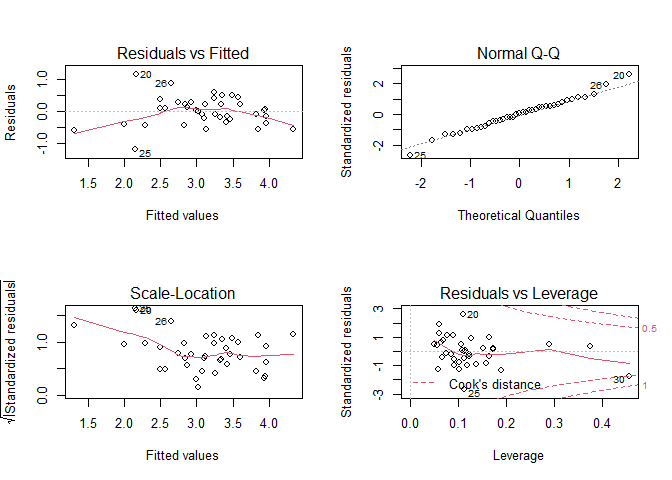par(mfrow = c(1, 1)) hist(res, main = "Histogram of residuals", xlab = "Residuals")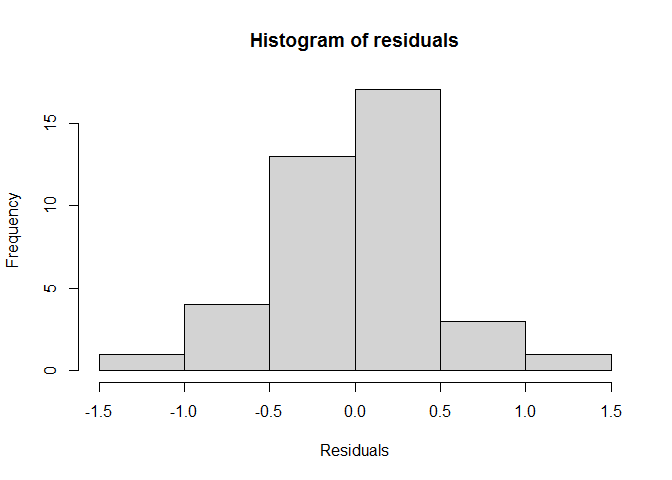bptest(mod2) ## ## studentized Breusch-Pagan test ## ## data: mod2 ## BP = 9.0755, df = 4, p-value = 0.05924 #### Question 60 Does a visual inspection of the residual plots and histogram indicate that the model assumptions appear to be satisfied? Explain your answer. Yes, a visual inspection of the residual plots and histogram indicate that the model assumptions appear to be satisfied. The regression line appears to be a good fit, because there does not seem to be any distinct patterns of residuals (in the Residuals vs Fitted graph). Also, residuals appear to be normally distributed as they follow a straight line quite well in the Normal Q-Q plot and the histogram of residuals is bell-shaped. Other plots also indicate that the model assumptions appear to be satisfied. #### Question 61 Also perform a Breusch-Pagan test for homogeneity of variance among the residuals. Use a 5% level of significance (you can include the code in your answer to Question 59). Comment on the results of the Breusch-Pagan test. Since p-value = 0.059 > 0.05, the null hypothesis of homoscedasticity cannot be rejected at a 5% level of significance. There is homogeneity of variance among the residuals. ### Part C Fit the model (same as in Part B, create the interaction term separately - do not use x1:x3 in code) $y = \beta_0 + \beta_1 x_1 + \beta_2 x_2 + \beta_3 x_3 + \beta_4 x_1 x_3 + \epsilon$ #### Question 62 Construct a 95% confidence interval for the mean gpa of all 7th-graders with Piers-Harris Children’s Self-Concept Score of 50, IQ of 105, and who are auditory learners. newdata <- data.frame(x1 = 50, x2 = 105, x3 = "Auditory", x1x3 = 50) predict(mod2, newdata, interval = "confidence") ## fit lwr upr ## 1 2.997526 2.696134 3.298919 #### Question 63 Enter the lower bound of the 95% CI (round to 3 decimal places). 2.696 #### Question 64 Enter the upper bound of the 95% CI (round to 3 decimal places). 3.299 #### Question 65 Write an interpretation for the interval in the context of the problem. We are 95% confident that for all 7th-graders with Piers-Harris Children’s Self-Concept Score of 50, IQ of 105, and who are auditory learners, the mean GPA is between 2.696 and 3.299. ### Part D Fit the model $y = \beta_0 + \beta_1 x_1 + \beta_2 x_2 + \beta_3 x_3 + \beta_4 x_1 x_3 + \epsilon$ #### Question 66 Interpret the value of $$\hat{\beta_2}$$ in the context of the problem. The value of $$\hat{\beta_2} = 0.033$$ shows that for each unit increase in IQ (x2), the GPA score is expected to increase by 0.033. ### Part E Fit the first-order logistic regression model to predict that a randomly selected student is an auditory learner from gpa, the Piers-Harris Children’s Self-Concept Score, and the IQ. #### Question 67 Use it to predict the probability of being an auditory learner for a 7th-grader with a 3.5 gpa, Piers-Harris Children’s Self-Concept Score of 50, and IQ of 105. mod_glm <- glm(x3 ~ y + x1 + x2, family = binomial, data = gpa7th) newdata_glm <- data.frame(y = 3.5, x1 = 50, x2 = 105) predict(mod_glm, newdata_glm, type = "response") ## 1 ## 0.7665911 #### Question 68 Report the probability to 4 decimal places. Answer: 0.7666 ## Problem 6 Scenario: A study was conducted to evaluate the quality of beef after storage times (STORAGE) of 10, 40, 80, and 120 days. The beef quality variables assessed were beefy aroma (BEEFY), bloody aroma (BLOODY), and a grassy aroma (GRASSY), which were all measured on a rating scale ranging from 0 to 15. Thirty samples were evaluated by several beef quality specialists and the average rating was obtained for this data file. You will need this file: beef.rda ### Part A #### Question 69 Use the Henze-Zirkler Multivariate Normality Test to test for multivariate normality among the three response variables: BEEFY, BLOODY, and GRASSY. Include a chi-square quantile plot in your analysis and use a 1% level of significance for each individual hypothesis test. library(MVN) load("beef.rda") mvn(beef[, -1], mvnTest = "hz", multivariatePlot = "qq")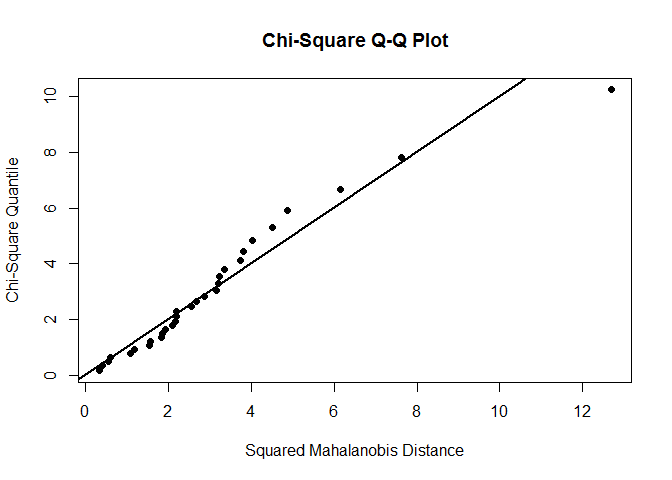##$multivariateNormality
##            Test        HZ   p value MVN
## 1 Henze-Zirkler 0.7762501 0.1296601 YES
##
## $univariateNormality ## Test Variable Statistic p value Normality ## 1 Shapiro-Wilk BEEFY 0.8791 0.0027 NO ## 2 Shapiro-Wilk BLOODY 0.8937 0.0059 NO ## 3 Shapiro-Wilk GRASSY 0.9054 0.0114 NO ## ##$Descriptives
##         n     Mean  Std.Dev   Median     Min      Max    25th      75th
## BEEFY  30 7.583343 3.703254 7.875000 2.25000 12.66670 3.56250 11.291700
## BLOODY 30 4.938889 1.992796 5.500000 1.66667  7.41667 3.06250  6.729167
## GRASSY 30 1.955555 1.457037 1.916665 0.00000  4.41667 0.58333  3.250000
##              Skew  Kurtosis
## BEEFY  -0.1221207 -1.680482
## BLOODY -0.3083830 -1.469143
## GRASSY  0.1412231 -1.577458

#### Question 70

According to this test, is there sufficient evidence to conclude that BEEFY, BLOODY, and GRASSY are not multivariate normal? Explain.

According to this test, there is no sufficient evidence to conclude that BEEFY, BLOODY, and GRASSY are not multivariate normal. The p-value = 0.13 > 0.01 and the chi-square quantile plot indicates that data are multivariate normal.

### Part B

Conduct Box’s M Test to test for equality of covariances. Use a 1% level of significance.

#### Question 71

Is there sufficient evidence to conclude that the covariance matrices are not equal at the 1% level of significance?

There is no sufficient evidence to conclude that the covariance matrices are not equal at the 1% level of significance, because p-value = 0.022 > 0.01.

#### Question 72

library(biotools)
## ---
## biotools version 4.0
boxM(beef[, -1], beef[, 1])
##
##  Box's M-test for Homogeneity of Covariance Matrices
##
## data:  beef[, -1]
## Chi-Sq (approx.) = 31.973, df = 18, p-value = 0.02215

#### Question 73

Based on the criteria of multivariate normality and equal covariance matrices, is it appropriate to proceed with MANOVA?

Yes.

### Part C

#### Question 74

Regardless of the outcomes of the previous hypothesis tests, conduct a MANOVA to determine if there are differences between the different storage times for the population mean vectors when beefy, bloody, and grassy aromas are considered together. Use the Wilk’s Lambda statistic and let $$\alpha = .05$$

res.man <- manova(cbind(BEEFY, BLOODY, GRASSY) ~ STORAGE, data = beef)
summary(res.man, test = "Wilks")
##           Df  Wilks approx F num Df den Df    Pr(>F)
## STORAGE    3 0.1269   8.6897      9  58.56 3.816e-08 ***
## Residuals 26
## ---
## Signif. codes:  0 '***' 0.001 '**' 0.01 '*' 0.05 '.' 0.1 ' ' 1

#### Question 75

State the null and alternative hypothesis.

$$H_0:$$ mean vectors are equal across the groups;

$$H_a:$$ at least two of the groups’ mean vectors differ.

#### Question 76

State the conclusion for the test.

Since p-value < 0.05, we reject the null hypothesis. There are differences between the different storage times for the population mean vectors.

#### Question 77

Round the p-value to four decimal places (enter 0 if P < 0.00005).

p-value = 0

### Part D

#### Question 78

Follow up with univariate ANOVAs and Tukey multiple comparisons on the response variables to see which population means differ. Use a 5% level of significance for each univariate ANOVA and each Tukey procedure. (We are temporarily ignoring the multiple comparisons problem.)

summary.aov(res.man)
##  Response BEEFY :
##             Df Sum Sq Mean Sq F value    Pr(>F)
## STORAGE      3 322.55 107.516  37.192 1.491e-09 ***
## Residuals   26  75.16   2.891
## ---
## Signif. codes:  0 '***' 0.001 '**' 0.01 '*' 0.05 '.' 0.1 ' ' 1
##
##  Response BLOODY :
##             Df Sum Sq Mean Sq F value    Pr(>F)
## STORAGE      3 91.744 30.5814  33.948 3.837e-09 ***
## Residuals   26 23.422  0.9008
## ---
## Signif. codes:  0 '***' 0.001 '**' 0.01 '*' 0.05 '.' 0.1 ' ' 1
##
##  Response GRASSY :
##             Df Sum Sq Mean Sq F value    Pr(>F)
## STORAGE      3 51.727 17.2422  45.563 1.713e-10 ***
## Residuals   26  9.839  0.3784
## ---
## Signif. codes:  0 '***' 0.001 '**' 0.01 '*' 0.05 '.' 0.1 ' ' 1
TukeyHSD(aov(BEEFY ~ STORAGE, data = beef))
##   Tukey multiple comparisons of means
##     95% family-wise confidence level
##
## Fit: aov(formula = BEEFY ~ STORAGE, data = beef)
##
## $STORAGE ## diff lwr upr p adj ## 40-10 -1.171283 -3.629593 1.2870267 0.5668196 ## 80-10 -6.212302 -8.807288 -3.6173169 0.0000033 ## 120-10 -7.881942 -10.400960 -5.3629235 0.0000000 ## 80-40 -5.041019 -7.391613 -2.6904251 0.0000189 ## 120-40 -6.710658 -8.977108 -4.4442085 0.0000001 ## 120-80 -1.669639 -4.083652 0.7443732 0.2537189 TukeyHSD(aov(BLOODY ~ STORAGE, data = beef)) ## Tukey multiple comparisons of means ## 95% family-wise confidence level ## ## Fit: aov(formula = BLOODY ~ STORAGE, data = beef) ## ##$STORAGE
##              diff       lwr        upr     p adj
## 40-10  -0.5138894 -1.886182  0.8584034 0.7351889
## 80-10  -3.2559536 -4.704542 -1.8073650 0.0000092
## 120-10 -4.1458363 -5.552018 -2.7396546 0.0000001
## 80-40  -2.7420641 -4.054227 -1.4299012 0.0000279
## 120-40 -3.6319468 -4.897138 -2.3667554 0.0000001
## 120-80 -0.8898827 -2.237447  0.4576821 0.2907963
TukeyHSD(aov(GRASSY ~ STORAGE, data = beef))
##   Tukey multiple comparisons of means
##     95% family-wise confidence level
##
## Fit: aov(formula = GRASSY ~ STORAGE, data = beef)
##
## \$STORAGE
##             diff        lwr      upr     p adj
## 40-10  0.6527761 -0.2366643 1.542217 0.2090224
## 80-10  2.3472207  1.4083298 3.286112 0.0000016
## 120-10 3.3472213  2.4358160 4.258626 0.0000000
## 80-40  1.6944446  0.8439769 2.544912 0.0000556
## 120-40 2.6944451  1.8744216 3.514469 0.0000000
## 120-80 1.0000005  0.1265874 1.873414 0.0203952

#### Question 79

From the univariate ANOVA tests, we can see that all the response variables (BEEFY, BLOODY, GRASSY) are highly significantly different among STORAGE times.

According to Tukey multiple comparisons tests, population mean scores for beefy and bloody aroma are different for storage times of 10 and 80, 10 and 120, 40 and 80, 40 and 120 days.

Population mean scores for grassy aroma are different for storage times of 10 and 80, 10 and 120, 40 and 80, 40 and 120 days and also 80 and 120 days.

#### Question 80

Match each nonparametric procedure with the parametric procedure with which it most closely corresponds.

• Wilcoxon Signed Rank - Paired data t-test
• Wilcoxon Rank Sum Test - Pooled t-test
• Kruskal-Wallis Test - One-way ANOVA
• Fisher’s Exact Test - Chi–square test for independence

#### Question 81

Which of the following could be used in multiple regression model selection?

• AIC
• PRESS
• VIF
• $$R^2_{Adj}$$
• ALL of the above

#### Question 82

A Type I error can best be described as:

1. not rejecting a false null hypothesis.
1. a sample that has not been chosen randomly.
1. using a two-sided alternative when a one-sided alternative would be sufficient.
1. concluding that the null hypothesis is false when in fact it is true.
1. mistakenly using a parametric procedure instead of a nonparametric procedure when the data is not normally distributed.

Answer: d) concluding that the null hypothesis is false when in fact it is true.

#### Question 83

Which of the following statements DOES NOT represent a model assumption of linear regression?

1. the standard deviation of the independent variable is normally distributed
1. the residuals are normally distributed
1. the variance of the error terms is constant at each value of x
1. the random error terms are independent
1. the mean of the random error terms is zero

Answer: a) the standard deviation of the independent variable is normally distributed

#### Question 84

Which of the following statements DOES NOT represent a model assumption of ANOVA?

1. the samples are independent
1. the population means are not equal
1. the samples are randomly selected
1. the population variances are all equal
1. the populations are normally distributed

Answer: b) the population means are not equal

#### Question 85

In linear regression, if the correlation coefficient between the response variable y and the explanatory variable x1 is zero, then

1. The scatterplot of x1 vs. y will be a perfectly straight line.
1. There is no linear relationship between x1 and y.
1. $$R^2$$ will be 1.
1. The observed values of y are equal to the predicted values.
1. We reject $$H_0: \beta_1 = 0$$

Answer: b) There is no linear relationship between x1 and y.

#### Question 86

For the following problems, match the scenario to the appropriate statistical procedure. Each procedure may be used more than once or not at all.

Scenario: A business manager has data for 36 variables collected for the employees in various positions within a very large organization. The manager would like to reduce the number of variables to a much smaller number of latent variables that will represent the shared variability present in the original 36 variables.

Procedure: Factor Analysis

Scenario: A random sample of American adults is collected to see if how systolic blood pressure is related to weight, height, age, and gender.

Procedure: Linear regression

Scenario: A manufacturing plant measures the weekly man-hours lost due to accidents for a random sample of its employees both before and after the installation of an elaborate safety program and would like to conduct a test to see if the loss of man-hours is lower after the program. The difference in loss of man-hours is not normally distributed.

Procedure: Wilcoxon signed rank test

Scenario: A clinical research study is designed to investigate how systolic blood pressure, cholesterol level, and whether or not a person is a smoker can predict the presence or absence of hypertension.

Procedure: Logistic regression

Scenario: Do elementary, middle, and high school students differ in the amount of time spent watching television per week? Sample data looks to be normally distributed with approximately equal variance.

Procedure: ANOVA

Scenario: Is the probability distribution of college students at a college football game such that there are equal proportions of Freshmen, Sophomores, Juniors, and Seniors?

Procedure: Chi-Square test for Goodness-of-fit

Scenario: Economists collect independent random samples from five different professional disciplines in order to compare the population variances of salaries in each.

Procedure: Levene test